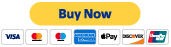Temp: Page is about math formulas and stuffs.

This paper provides a method using periodic functions to check for primality, count factors, list factors, calculate the exact prime distribution, and determine the Nth prime.  It describes this method in a straightforward manner, from one equation to the next, using graphs between each key step to help quickly visualize the reasoning and result of each maneuver.  It concludes with brief afterthoughts and ongoing questions about the technique.  The last 4 pictures are examples of using the Factor Tagging Function, to factor the numbers 1 through 10, 12, 212, 523, 1111, and list the specific factors of 212.© 2017- 2020 by Thoughtfarm. Proudly created with Wix.com